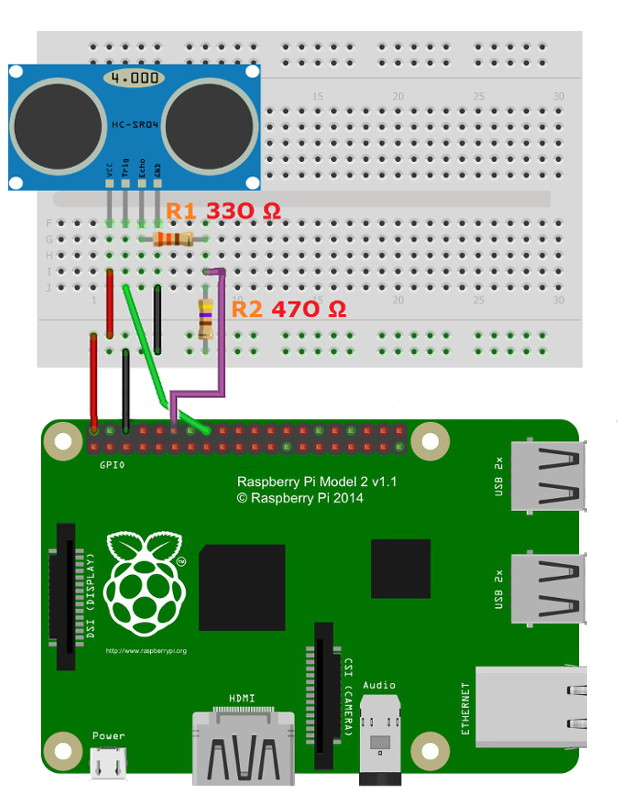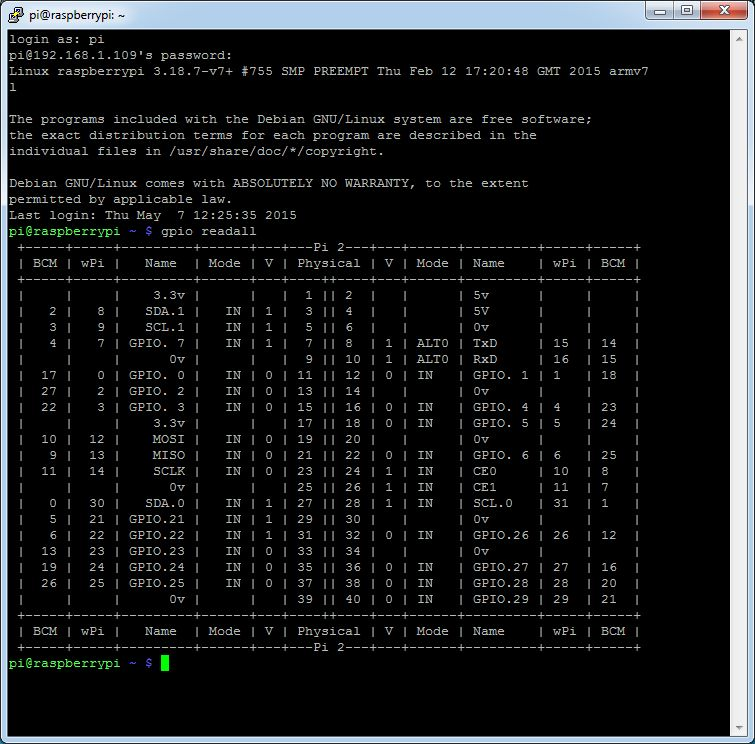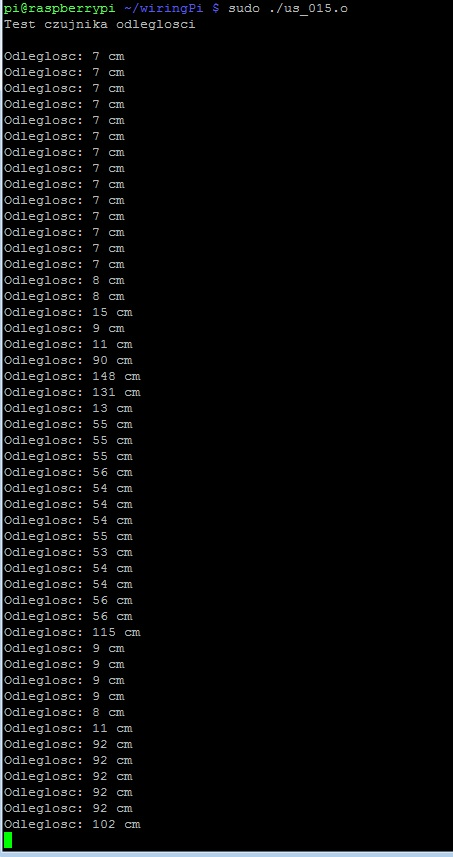# Distance measurement using Raspberry Pi and HC-SR04 or US-015

## Distance measurement using the Raspberry Pi and the sensor HC-SR04 US-015

Presents sensors provide distance measurement using ultrasound. The sensor sends out a wave, which after reflection from the obstacle, it returns to him. Computing the time between transmission and reception of the pulse can determine the distance of the sensor from the object.

#### Connect to the Raspberry Pi

You need to connect both devices at the table

GPIO Raspberry PiDistance sensor
5 VVCC
GNDGND

GPIO 4

Trig

GPIO 1

EchoThe scheme of connection of ultrasonic sensor with the Raspberry Pi.

#### Service

In order to use GPIO pins in the Raspberry Pi we need a library that permits us to do it. To this end, we use wiringPi. Description how to download and install find here. Simply copy the following command. In the end, to validate the installation:

After this command we should see the following table:Now we will write a simple program. Create a file us_015.cpp

• nano us_015.cpp

The file should contain the following programs:

```#include
#include
#include <sys/time.h>
using namespace std;

int CM;
long TIME;
int trig = 4;
int echo = 1;
void pomiar_odleglosci();
int pulseIn(int pin, int level);

int main (void)
{
wiringPiSetup ();

pinMode(trig, OUTPUT);
pinMode(echo, INPUT);

cout <<"Test sensor"<<endl<<endl;

while(1)
{
pomiar_odleglosci(); //measure the distance
cout<<"Distance: "; //display results on the screen in a loop every 200 MS
cout<<CM<<" cm"<<endl;
delay(200);
}
return 0 ;
}

void pomiar_odleglosci ()
{
digitalWrite(trig, HIGH); //setting the high position for 10 uS - impul\$
delayMicroseconds(10);
digitalWrite(trig, LOW);
TIME = pulseIn(echo, HIGH);
CM = DURATION / 58; //width of the reflected pulse in the us split p\$
}

int pulseIn(int pin, int level) //function to measure the time to reach the specified \$
{
struct timeval tn, t0, t1;
long micros;
gettimeofday(&t0, NULL);
micros = 0;

{
gettimeofday(&tn, NULL);

if (tn.tv_sec > t0.tv_sec) micros = 1000000L; else micros = 0;

micros += (tn.tv_usec - t0.tv_usec);

if (micros > 10000) return 0;
}

gettimeofday(&t1, NULL);

{
gettimeofday(&tn, NULL);

if (tn.tv_sec > t0.tv_sec) micros = 1000000L; else micros = 0;

micros = micros + (tn.tv_usec - t0.tv_usec);

if (micros > 10000) return 0;
}

if (tn.tv_sec > t1.tv_sec) micros = 1000000L; else micros = 0;
micros = micros + (tn.tv_usec - t1.tv_usec);

return micros;
}

```

Then save the changes to the file and close it. To compile the file you created, use the command:

• g++ -Wall -o us_015.on us_015.cpp -lwiringPi

#### The program

To calculate the distance to the object, the response time is common to 58 (according to the documentation). Why so much? This value is derived by the formula:

TIME / [1/(0.34 / 2 )/10]

• the value in square brackets is equal to 58:
• 0.34 - wave velocity in m/MS (340 m/sec) divided by 2 (since the wave has come a long way in the two sides).
• In addition, all safely by 10 to change millimetres to centimeters

The program is run using the following command:

• sudo ./us_015.on

The result of the program can be viewed on the monitor:The screen of the monitor.